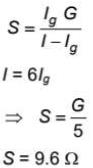Courses

# Sample Test: Physics

## 20 Questions MCQ Test NEET Mock Test Series | Sample Test: Physics

Description
This mock test of Sample Test: Physics for NEET helps you for every NEET entrance exam. This contains 20 Multiple Choice Questions for NEET Sample Test: Physics (mcq) to study with solutions a complete question bank. The solved questions answers in this Sample Test: Physics quiz give you a good mix of easy questions and tough questions. NEET students definitely take this Sample Test: Physics exercise for a better result in the exam. You can find other Sample Test: Physics extra questions, long questions & short questions for NEET on EduRev as well by searching above.
QUESTION: 1

### In an n-p-n transistor, 108 electrons are emitted from the emitter in 10-4 s. If  2% of electrons are lost in the base. Then the current gain in common-emitter configuration mode is

Solution: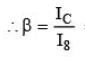= (98/100) / (2/100)
= 98/2
= 49

QUESTION: 2

### Electromagnetic radiations of intensity I is incident normally on a perfectly reflecting surface. If speed of light is C, then radiation pressure exerted on the surface is

Solution:

Let no. of photons falling per second per unit area = n
So, I = nhf
Pressure = Force / Area = 2n(h / λ) = 2nhf / c = 2I / C

QUESTION: 3

### A simple microscope has a focal length of 2.5 cm. Maximum angular magnification of it will be (Given that least distance of clear vision = D = 25 cm)

Solution: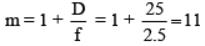QUESTION: 4

Suppose θ is polarising angle for a transparent medium and speed of light in that medium is v, then according to Brewster law.

Solution: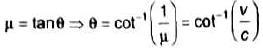QUESTION: 5

A convex lens made up of glass (μ = 1.5) has 20 cm focal length in air (μ = 1). Then its focal length in water  (μ = 1.33) will be:

Solution: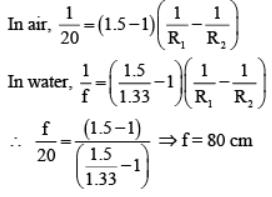QUESTION: 6

An eye specialist prescribes spectacles having a combination of a convex lens of focal length 40 cm in contact with a concave lens of focal length 25 cm. The power of this lens combination is

Solution: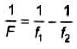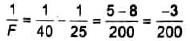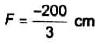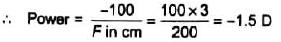QUESTION: 7

A nucleus of mass number 220, initially at rest emits an α -particle. If the Q value of the reaction is 5 MeV, then the energy of emitted α -particle will be

Solution: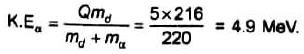QUESTION: 8

A sample of radioactive material has a mass m, decay constant λ and molecular weight M. If avogadro’s constant is NA. Then initial activity of the sample is

Solution: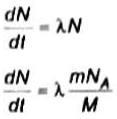QUESTION: 9

Two nucleons are at a separation of 1 fm. The net force between them is F1 if both are neutrons, F2 if both are protons, and F3 if one is a proton and the other is a neutron.

Solution:

Nuclear forces will be same all three cases but total force F2 will be smaller because of electrostatic repulsion between two protons.

QUESTION: 10

Suppose the potential energy between electron and proton at a distance r is vary as U ∝ r2. Assuming Bohr's model of quantization of angular momentum and circular orbits, radius of the nth allowed orbit is proportional to

Solution: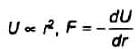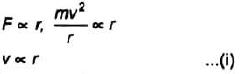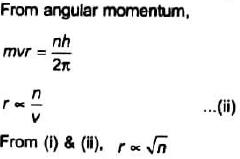QUESTION: 11

The ionization potential of hydrogen atom is − 13.6 V. Photons of energy 12.75 eV are made to incident on sample of H atoms at ground state. How many spectral lines are expected in emitted radiation?

Solution:

E=E1/n2
Energy used for excitation is 12.75 eV
ie, (-13.6+12.75)eV = -0.85eV image
Energy levels of H-atom
The photons of energy 12.75eV can excite the fourth level of H-atom. Therefore, six lines will be emitted. [ n (n−1) /2 lines].

QUESTION: 12

de-Brogile wavelength associated with a proton accelerated from rest by 10 kV is λ. The de-Brogile wavelength associated with a 10 keV neutron is

Solution: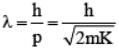, where k is kinetic energy of particle. According to given data, kinetic energy of proton is 10keV which is same as that of neutron. Masses are also same.

QUESTION: 13

A body of mass 6 kg is lowered down with help of a string with downward acceleration g/4. The work done by the string on the body in first 3s after start of the motion is (g = 10 m/s2)

Solution: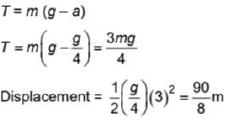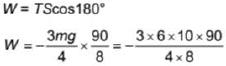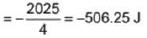QUESTION: 14

Light from three non-coherent sources, having same intensity, interfere at a location and produce resultant intensity 6I. If one of the three sources is switched off, then the resultant intensity at same point will be

Solution:

In case of non-coherent sources I net = ∑l
Let intensity from one source by l
⇒ 3lo = 6l
⇒ lo = 2I
If one source is switched off
Net intensity = 2Io = 4I

QUESTION: 15

Figure shows a flywheel of radius 10 cm. Its moment of inertia about the axis of rotation is 0.4 kg m2. A massless string passes over the flywheel and a mass of 2 kg attached at its lower end. Angular acceleration of flywheel in (rad/s2) is nearly (g = m/s2)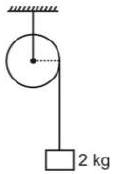Solution: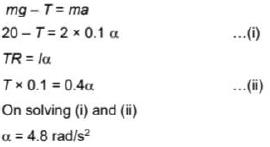QUESTION: 16

Radius of a planet is three times the radius of the earth and it has same average density as that of the earth. The ratio of escape speeds from surface of the planet and that from the surface of earth is

Solution: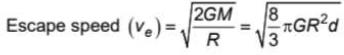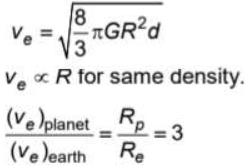QUESTION: 17

Heat is supplied to a certain sample of a substance in solid state at a constant rate. The variation of temperature is plotted against time as shown in the figure. Choose the correct statement regarding the sample.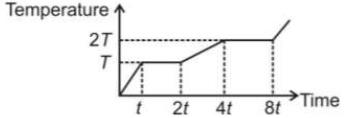Solution:

Since time required for same temperature increase is larger in liquid state than solid state, the specific heat capacity is higher in liquid state. Since in phase transition, the heat required is less in fusion than evaporation, hence latent heat of fusion is less.

QUESTION: 18

A simple pendulum has a time period T in vacuum. Its time period when it is completely immersed in a nonviscous liquid of density one-fourth density of material of bob is

Solution: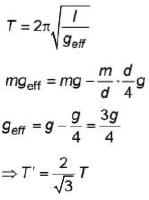QUESTION: 19

A car is moving on a road with a speed 40 m/s, blows a horn of frequency 200 Hz. The apparent wavelength of sound as observed by a detector on the road behind the car will be (Speed of sound in air is 340 m/s)

Solution: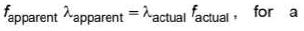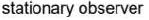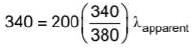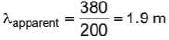QUESTION: 20

The resistance of a galvanometer coil is 48 Ω . The resistance of shunt required to convert it into an ammeter of range 6 times as that of galvanometer is

Solution: